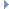Arduino Helpers master Utility library for Arduino
Math Types

## Detailed Description

Vector and Quaternion types with the necessary operators and functions.Collaboration diagram for Math Types:

## Classes

struct  Quaternion
Type for quaternions of floating point numbers. More...

struct  EulerAngles
Struct for Euler angles of floating point numbers. More...

struct  Vec2f
Type for 2D vectors of floating point numbers. More...

struct  Vec3f
Type for 3D vectors of floating point numbers. More...

## Functions

Quaternion operator* (float lhs, Quaternion rhs)
Scalar multiplication. More...

Print & operator<< (Print &os, Quaternion e)
Printing. More...

Print & operator<< (Print &os, EulerAngles e)
Printing. More...

Vec2f operator* (float lhs, Vec2f rhs)
Scalar multiplication. More...

Vec3f operator* (float lhs, Vec3f rhs)
Scalar multiplication. More...

Print & operator<< (Print &os, Vec2f v)
Printing. More...

Print & operator<< (Print &os, Vec3f v)
Printing. More...

## ◆ operator*() [1/3]

 Quaternion operator* ( float lhs, Quaternion rhs )
related

Scalar multiplication.

Definition at line 278 of file Quaternion.hpp.

## ◆ operator<<() [1/4]

 Print & operator<< ( Print & os, Quaternion e )
related

Printing.

Definition at line 28 of file Quaternion.cpp.

## ◆ operator<<() [2/4]

 Print & operator<< ( Print & os, EulerAngles e )
related

Printing.

Definition at line 32 of file Quaternion.cpp.

## ◆ operator*() [2/3]

 Vec2f operator* ( float lhs, Vec2f rhs )
related

Scalar multiplication.

Definition at line 128 of file Vector.hpp.

## ◆ operator*() [3/3]

 Vec3f operator* ( float lhs, Vec3f rhs )
related

Scalar multiplication.

Definition at line 242 of file Vector.hpp.

## ◆ operator<<() [3/4]

 Print & operator<< ( Print & os, Vec2f v )
related

Printing.

Definition at line 32 of file Vector.cpp.

## ◆ operator<<() [4/4]

 Print & operator<< ( Print & os, Vec3f v )
related

Printing.

Definition at line 38 of file Vector.cpp.